# AP Board 5th Class Maths Solutions 1st Lesson Let’s Recall

Andhra Pradesh AP Board 5th Class Maths Solutions 1st Lesson Let’s Recall Textbook Exercise Questions and Answers.

## AP State Syllabus 5th Class Maths Solutions Chapter 1 Let’s Recall

Question 1.
Sai is studying 5th class and his sistef Valli is studying 3rd class. In summer holidays, Sai and Valli wanted to go to Bangalore with their grand-father Nagayya. They reached Vijayawada railway-station.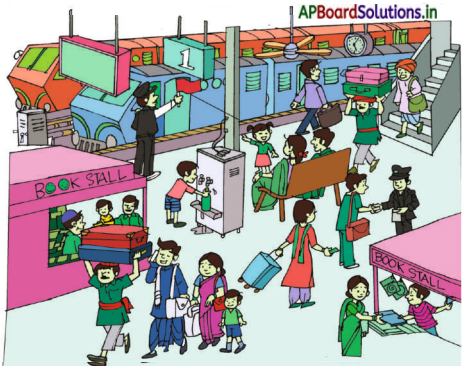Observe the picture and answer the following questions.

1. What is the platform number ?
The platform number is 1.

2. How many persons are sitting on the bench?
Two members are sitting on the bench.

3. What is the time shows in the clock?
The time shows in the clock is 5 : 05

4. How many blades are there to the ceiling fan ?
4 blades are there to the ceiling fan.

5. How many children are there in the picture ?
4 children are there in the picture.

6. How many persons can you see in the
picture?
20 persons are there in the picture.Exercise 1:

Question 1.
Write the following numbers in words.
a) 9 –
Nine

b) 37 –
Thirtyseven

c) 267 –
Two hundred and sixty seven

d) 607 –
Six hundred and seven

e) 5298 –
Five thousand and two hundred ninety eight

f) 1307 –
One thousand three hundred and seven

g) 42689 –
Forty two thousand six hundred and Eighty Nine

h) 52006 –
Fifty two thousand and six.Question 2.
Write the expanded form for the following numbers.
a) 62 =
60 + 2

b) 30 =
30 + 9

c) 792 =
700 + 90 + 2

d) 308 =
300 + 00 + 8

e) 3472 =
3000 + 400 + 70 + 2

f) 9210 =
9000 + 200 + 10 + 0

g) 61287 =
60000 + 1000 + 200 + 80 + 7

h) 20508 =
20000 + 0 + 500 + 00 + 8.Question 3.
Write the place values of the underlined digits in the following numbers.
a) 48
4 is in tens place = 40

b) 63
3 is in units place = 3

c) 834
8 is in hundredth place = 800

d) 607
0 is in tens place = 0

e) 2519
2 is in thousands place = 2000

f) 6920
2 is in tens place = 20

g) 12453
4 is in hundreds place = 400

h) 52146
5 is in ten thousands place = 50,000.Question 4.
Find the sum of the place values of 6 in the following numbers.

a) 266
In 266, Sum of place values of 6 is = 60 + 6 = 66

b) 616
In 616, Sum of place values of 6 is = 600 + 6 = 606

c) 665
In 665, Sum of place values of 6 is = 600 + 60 = 660

d) 6236
In 6236, Sum of place values of 6 is = 6000 + 6 = 6006

e) 64,624
In 64,624, Sum of place values of 6 is = 60,000 + 600 = 60,600

f) 67,426
In 67,426, Sum of place value of 6 is = 60,000 + 6 = 60,006

g) 86,216
In 86,216, Sum of place value of 6 is = 6000 + 6 = 6006.

Question 5.
Write the predecessor and successor for the following numbers.

a) 9
Predecessor of 9 is = 8
Successor of 9 is = 10

b) 99
Predecessor of 99 is = 98
Successor of 99 is = 100

c) 539
Predecessor of 539 is = 538
Successor of 539 is = 540

d) 621
Predecessor of 621 is = 620
Successor of 621 is = 622

e) 4001
Predecessor of 4001 is = 4000
Successor of 4001 is = 4002

f) 3210
Predecessor of 3210 is = 3209
Successor of 3210 is = 3211

g) 10000
Predecessor of 10000 is = 9999
Successor of 10000 is = 10001.Question 6.
Fill the following table: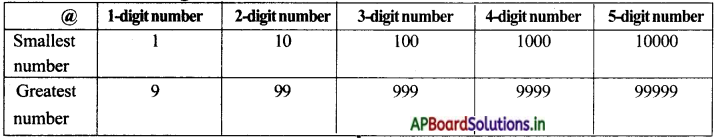Question 7.
Write the suitable numbers for the following.

a) 10 + 2 =
12

b) 200 + 30 + 5 =
235

c) 4000 + 500 + 70 + 4 =
4574

d) 10000 + 3000 + 500 + 50 + 6 =
13556

e) 50000 + 2000 + 800 + 50 + 7 =
52857

f) 30.000 + 500 + 8 =
30.508.Question 8.
Write the greatest 4-digit number by using 7, 6, 5 and 2
7,652

Question 9.
Write the smallest 4-digit number by using 2, 0. 8 and 7
2078

Question 10.
How many 100 s are there in 1,000 ?
Ten 100’s are there in 1000

TextBook Page No.8:

Nagayya tooks his bank-pass book from his handbag to see his bank transactions. Sai was very curious to see the bank-pass book. Grand-father gave his pass-book to Sai. He explained all the entries in the Pass Book. (T.P-8)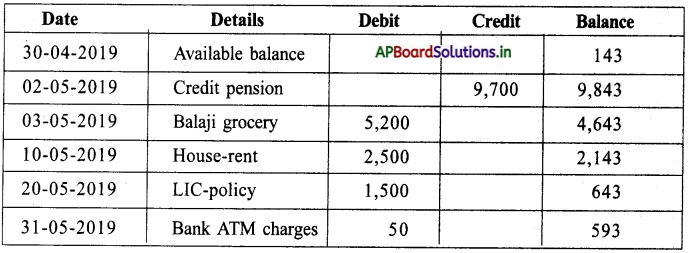Question 1.
When did Nagayya have the hghest balance amount ? How much ?
On 02-05-2019, Nagayya have the highest balance amount i.e. 9843.

Question 2.
When did he have the lowest balance amount ? How much ?
On 30-04-2019, Nagayya have the lowest balance amount i.e. 143.

Question 3.
Compare 9,843 and 143 with suitable symbols (< or = or >)
9,843 > 143.

Question 4.
Write the amounts in the balance column in ascending order.
143 < 593 < 643 < 2,143 < 4,643 < 9,843.

Question 5.
Write the amounts in the balance column in descending order.
9,843 > 4,643 > 2,143 > 643 > 593 > 143.Exercise 2:

Question 1.
Round off the following numbers to the nearest tens.

a) 32
Nearest tens to 32 is = 30

b) 78
Nearest tens to 78 is = 80

c) 123
Nearest tens to 123 is = 120

d) 485
Nearest tens to 485 is = 490

e) 2,546
Nearest tens to 2,546 is = 2550

f) 5,814
Nearest tens to 5,814 is = 5200

g) 25,796
Nearest tens to25,796 is = 25,800.

Question 2.
Round off the following numbers to the nearest hundreds.

a) 312
Nearest hundreds to 312 is 300

b) 956
Nearest hundreds to 956 is 1000

c) 123
Nearest hundreds to 123 is 100

d) 485
Nearest hundreds to 485 is 500

e) 2546
Nearest hundreds to 2546 is 2500

f) 5814
Nearest hundreds to 5814 is 5800

g) 796
Nearest hundreds to 796 is 800.Question 3.
Round off the following numbers to the nearest thousands.
a) 5264
Nearest thousands to 5264 is 5000

b) 7532
Nearest thousands to 7532 is 8000

c) 1234
Nearest thousands to 1234 is 1000

d) 4850
Nearest thousands to 4850 is 5000

e) 25463
Nearest thousands to 25,463 is 25,000

f) 5014
Nearest thousands to 5014 is 5000

g) 95150
Nearest thousands to 95,150 is 95,000

Question 4.
Use symbols (<, > or =) between the numbers.

a) 9 ___ 5
>

b) 21 ___ 39
<

c) 405 ___ 504
<

d) 1565 ___ 1565
=

e) 12578 ___ 25178
<

f) 90507 ___ 70503
>

g) 42179 ___ 42179
=

h) 81456 ___ 65899
>Question 5.
Write these numbers in ascending order.

a) 2, 1, 5, 9, 7
Ascending order: 1, 2, 5, 7, 9

b) 27, 46, 10, 29, 72
Ascending order: 10, 27, 29, 46, 72

c) 402, 204, 315, 351, 610
Ascending order : 204, 315, 351, 402, 610

d) 3725, 7536, 7455, 7399, 2361.
Ascending order: 2361, 3725, 7399, 7455, 7536

e) 25478, 25914, 25,104, 25072
Ascending order : 25072, 25104, 25478, 25914.

f) 46202, 10502, 60521, 81134
Ascending order: 10502, 46202, 60521, 81134

Question 6.
Write these Numbers in descending order.

a) 3, 8, 4, 2, 1
Descending order: 8, 4, 3, 2, 1

b) 97, 69, 96, 79, 90
Descending order: 97, 96, 90, 79, 69

c) 205, 402, 416, 318, 610
Descending order: 610, 416, 402, 318, 205

d) 8016, 916, 10219, 41205, 2430
Descending order: 41205, 10219, 8016, 2430, 916

e) 57832, 57823, 57830, 57820, 57825
Descending order: 57832, 57830, 57825, 57823, 57820

f) 16342, 86620, 46241, 64721, 46820
Descending order: 86620, 64721, 46820, 46241, 16342.Exercise 3:

Question 1.
Do the following.
a) 4 + 6 =
10

b) 9 + 5 =
14

c) 58 + 69 =
127

d) 45 + 27 =
72

e) 143 + 235 =
378

f) 539 + 709 =
1248

g) 2,658 + 5,131 =
7789

h) 2,056 + 8,997 =
11053

Question 2.
a) 8 – 5 =
3

b) 72 – 36 =
36

c) 82 – 37 =
45

d) 798 – 527 =
271

e) 850 – 456 =
394

f) 6527 – 2314 =
4213

g) 4526 – 2398 =
2128

h) 4005 – 2589 =
1416Question 3.
To get 5629, how much should we add to 1058?
Difference between 5629 and 1058 is = 4571
To get 5629, we add 4571 to 1058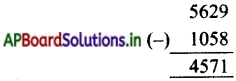Question 4.
To get 1250, how much should we subtract from 9658?
Difference between 1250 and 9658 is = 8408
∴ To get 1250, we subtract 8408 from 9658 =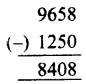Question 5.
Amount with Mounika = ₹ 5270
Radhika has ₹ 550 more than Mounika
Amount with Radhika = ₹ 5270 + ₹ 550 = ₹ 5820.
∴ Total money with Mounika and Radhika = ₹ 5270 + ₹ 5820 = ₹ 11,090.

Question 6.
Kohli made 120 runs in a match. Rohit made 65 runs less than Kebli In the same match. Find the total nw.s made by Kobil and Rohit.
Runs scored by Kohil = 120
Rohit made 65 runs less than Kohli.
Runs scored by Rohit = 120 – 65 = 55
∴ Total runs scored by Kohli and Rohit = 120 + 55 = 175 Runs.Exercise 4:

Question 1.
Do the following.

a) 8 × 2 =
16

b) 24 × 8 =
24 × 8 = 192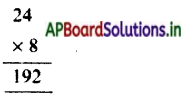c) 68 × 56 =
68 × 56 = 3808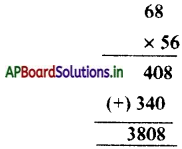d) 386 × 7 =
386 × 7 = 2702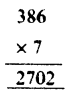e) 951 × 15 =
951 × 15 = 14265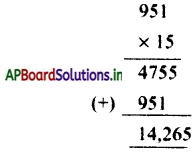f) 253 × 14 =
253 × 14 = 3542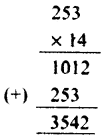g) 9685 × 20 =
9685 × 20 = 1,93,700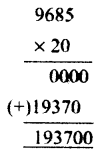Question 2.
Do the following:

a) 6 ÷ 2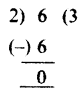b) 68 ÷ 7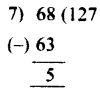c) 85 ÷ 3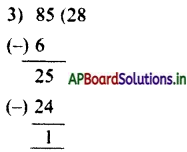d) 508 ÷ 4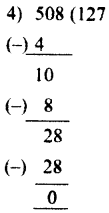e) 599 ÷ 13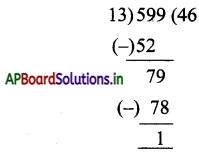f) 786 ÷ 16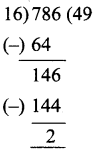g) 9501 ÷ 30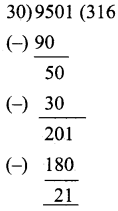Question 3.
In an apple box, there are 8 crates and in each crate there are 15 apples. how many apples are there in the box.
Number of crates in a box = 8
Number of apples in each crate = 15
Number of apples in the bos totally = 15 × 8 = 120

Question 4.
Product of two numbers is 560. One of the numbers ¡s 10. Find the other number.
Product of two numbers = 560
One number be = 10
Second number = 560 ÷ 10 = 56

Question 5.
If 45000 are distributed equally among 20 old age pensioners, then how much amount will each get?
Total amount = 45,000
Number of pensioners =20
Amount will each pensioners one get = 45000 ÷ 20 = 2,250.Question 6.
The cost of I dozen books is 840. What is the cost of I book? (1 Dozen = 12)
Number of books per dozen = 12
Cost of 1 dozen books = 840
Cost of 1 book = 840 ÷ 12 = ₹ 70

Question 7.
Complete the table.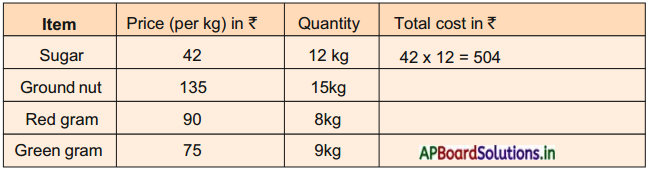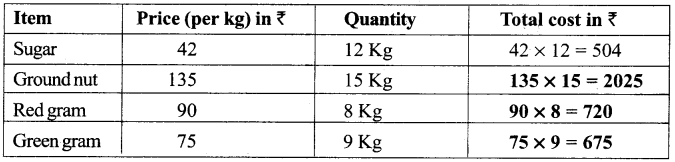Question 8.
Complete the table.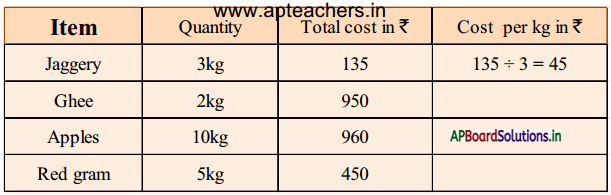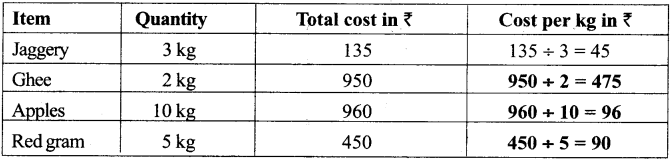Do these: (TextBook Page No.20)

Question 1.
Shade and write the fraction in boxes given below.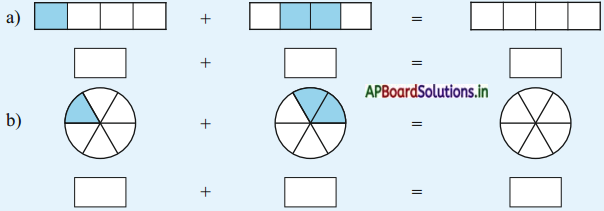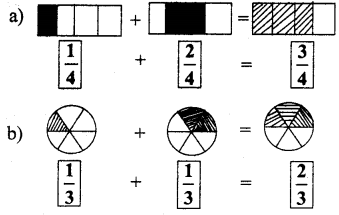Question 2.
Find the difference.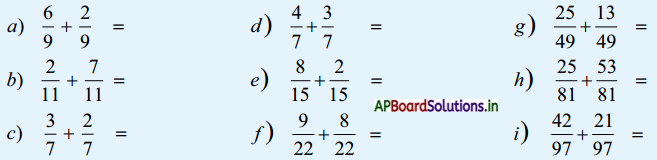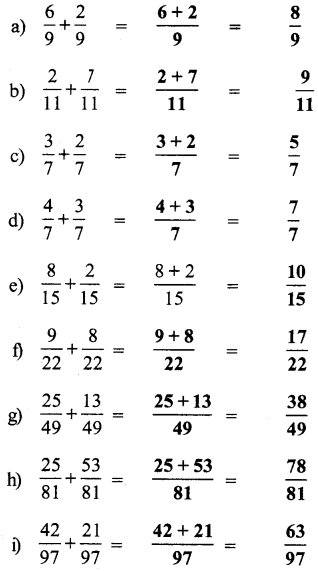Do these: (TextBook Page No.22)

Question 1.
Shade and write the fraction in boxes given below.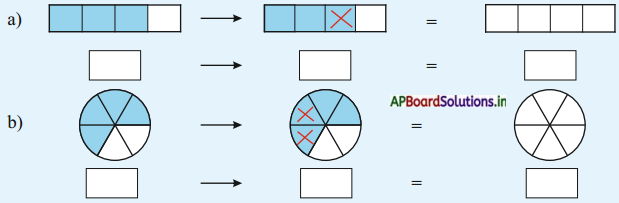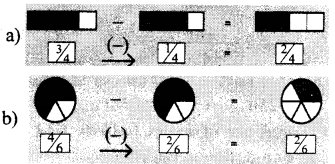Question 2.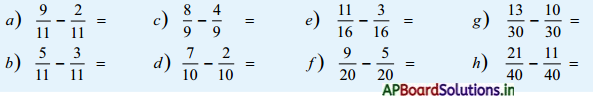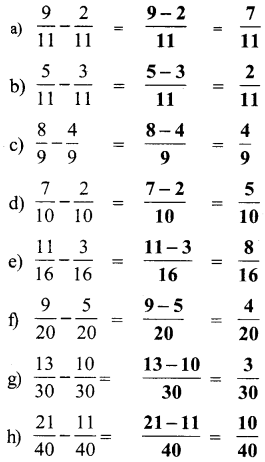Exercise 5:

Question 1.
Do the following:

a) 4578 + 121 =
4699

b) 897 + 9547 =
10444

c) 9897 + 6027 =
15924

d) 5240 + 253 + 32 + 5 =
5530

Question 2.
Giri received ₹ 2250 towards old age pension and ₹ 9500 under Rythu bharosa scheme. How much amount did he get
Giri received amount towards old age pension on = ₹ 2250
Amount received towards = ₹ 9500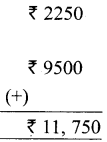Rythu bhrosa Scheme Total amount he get = ₹ 11,750.

Question 3.
In a village, the number of females is 250 more than that of males. If the number of males is 1590, find the population in the village.
Number of males =1590
The number of females is 250 more than that of males.
Number of females = 250 + 1590 = 1840
The population of the village = 1590 + 1840 = 3430.Question 4.
Do the following.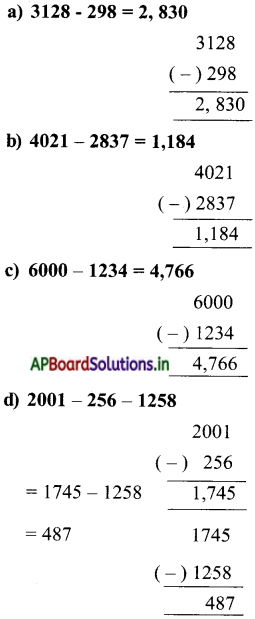Question 5.
Sum of two numbers is 7680. One of the two numbers is 2519. Find the other number.
One of the two numbers = 2519
Sum of two numbers = 7680
Other number = 7680 – 2519 = 5161.

Question 6.
Seetha has ₹ 6500. Raju has ₹ 155 less than Seetha’s amount. Find the amount with Raju.
Amount with Seetha = ₹ 6500
Raju has ₹ 155 less than Seetha’s amount
∴ Amount with Raju = ₹ 6500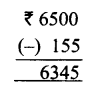Question 7.
Do the following.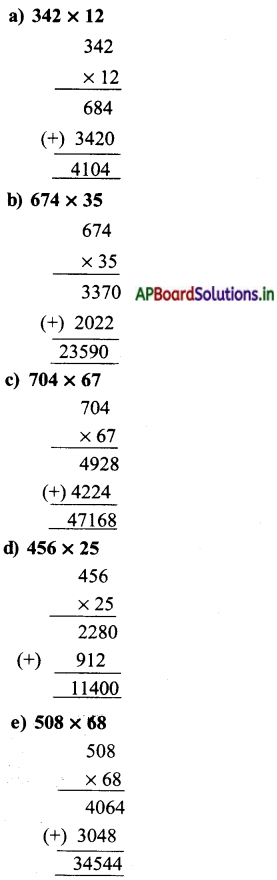Question 8.
Vijaya has ₹ 685, Karthik has 13 times more than Vijaya’s amount. Find the amount with Karthik.
Amount at Vïjaya = ₹ 685
Karthik has 13 times more than Vijaya’s Amount.
Amount at karthik = 13 × ₹ 685 = ₹ 8905.

Question 9.
The cost of a fan is ₹ 685 and a table is ₹ 2250. Find the total cost of 2 fans and 3 tables.
Cost of fan = ₹ 685
Cost of 2 fans = 2 × 685 = ₹ 1370
Cost of a table = ₹ 2250
Cost of 3 tables = 3 × 2250 = ₹ 6750
∴ Total cost of 2 fans + 3 tables = 1370 + 6750 = ₹ 8120.Question 10.
The cost of a paint bucket was 750. Lalitha wanted to paint her house, so she bought 5 buckets. How much amount did she pay for 5 buckets of paint?
Cost of a paint bucket = ₹ 750
Number of buckets required = 5
Amount did lalitha pay for 5 buckets of paint 5 × ₹ 750 = ₹ 3750.

Question 11.
A pair of shoes costs ₹ 250. A donar wants to donate shoes for 32 children in a school. Find how much amount will he require to buy shoes?
Cost of pair of shoes = ₹ 250
Number of children = 32
Total amount required to buy shoes = 32 × 250 = ₹ 8000

Question 12.
There are 105 words on each page of a book on an average. If the book contains 53 pages, find how many words are there in the book?
Number of words on each page of a book = 105
Number of pages = 53
Totally number of words are there in the book = 105 × 53 = 5565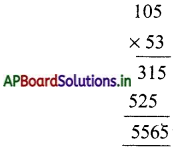Total number of words in the book = 5565 words.Question 13.
Do the following: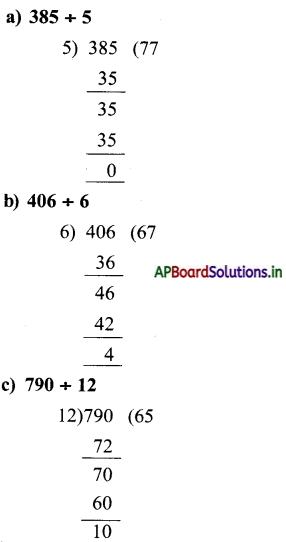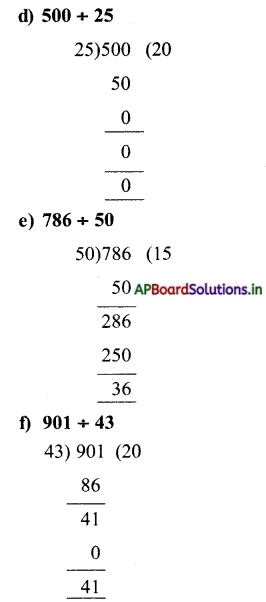Question 14.
Product of two numbers is 980. One of them is 5. Find the number.
Product of two numbers = 980
One number be = 5
Second number = 980 ÷ 5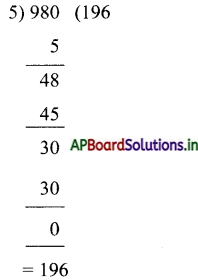Question 15.
If 125 chocolates were distributed equally among 25 members, find how many chocolates will each get?
Total chocolates = 125 Nos.
Members 25 Nos.
Each member get = 5 Nos.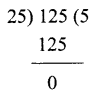Question 16.
a) $$\frac{3}{10}+\frac{4}{10}$$
$$\frac{3+4}{10}=\frac{7}{10}$$

b) $$\frac{4}{8}+\frac{3}{8}$$
$$\frac{4+3}{8}=\frac{7}{8}$$

c) $$\frac{7}{8}-\frac{2}{8}$$
$$\frac{7-2}{8}=\frac{5}{8}$$

d) $$\frac{4}{9}-\frac{1}{9}$$
$$\frac{4-1}{9}=\frac{3}{9}$$

Question 17.
Ravi read $$\frac{1}{4}$$th of the pages in a book. How much part is yet to be completed in the book ?
$$1-\frac{1}{4}=\frac{4-1}{4}=\frac{3}{4}$$ part to be completed by Ravi.Total Property distributed to both children = $$\frac{2}{7}+\frac{3}{7}=\frac{5}{7}$$